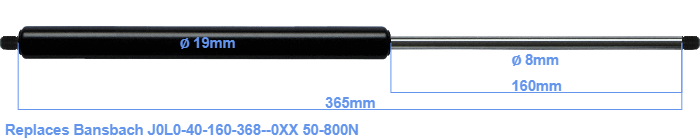# Replacement for Bansbach J0L0-40-160-368–0XX 50-800N

\$32.89

Replacement gas spring for the Bansbach J0L0-40-160-368--0XX 50-800 Newton. Thread: M8. Brand: Hahn.
 Force Choose an option50 Newton60 Newton80 Newton100 Newton120 Newton140 Newton150 Newton160 Newton180 Newton200 Newton220 Newton240 Newton250 Newton260 Newton280 Newton300 Newton320 Newton340 Newton350 Newton360 Newton380 Newton400 Newton420 Newton440 Newton450 Newton460 Newton480 Newton500 Newton520 Newton540 Newton550 Newton560 Newton580 Newton600 Newton620 Newton640 Newton650 Newton660 Newton680 Newton700 Newton720 Newton740 Newton750 Newton760 Newton780 Newton800 NewtonClearThe bore of the house of this replacement gas spring is 19 mm. The diameter of the rod totals 8 mm. The stroke (the retracting part) has a length of 160 mm. In total, the length is 365 millimeter. Attention: this is the length between the centers of the mounting parts. The distance from thread to thread (without mounting parts) is 365 mm. The force of this replacement gas spring is 50-800 Newton. This is not an original gas spring from Bansbach, but a so called replacement gas spring. This gas spring was produced by Stabilus subsidiary Hahn with the brand Stabilus Industry Line. The gas spring can be used without problems as a replacement, because the dimensions and force equal.